# Geodesic Bending Invariants for Shapes¶

Important: Please read the installation page for details about how to install the toolboxes. $\newcommand{\dotp}{\langle #1, #2 \rangle}$ $\newcommand{\enscond}{\lbrace #1, #2 \rbrace}$ $\newcommand{\pd}{ \frac{ \partial #1}{\partial #2} }$ $\newcommand{\umin}{\underset{#1}{\min}\;}$ $\newcommand{\umax}{\underset{#1}{\max}\;}$ $\newcommand{\umin}{\underset{#1}{\min}\;}$ $\newcommand{\uargmin}{\underset{#1}{argmin}\;}$ $\newcommand{\norm}{\|#1\|}$ $\newcommand{\abs}{\left|#1\right|}$ $\newcommand{\choice}{ \left\{ \begin{array}{l} #1 \end{array} \right. }$ $\newcommand{\pa}{\left(#1\right)}$ $\newcommand{\diag}{{diag}\left( #1 \right)}$ $\newcommand{\qandq}{\quad\text{and}\quad}$ $\newcommand{\qwhereq}{\quad\text{where}\quad}$ $\newcommand{\qifq}{ \quad \text{if} \quad }$ $\newcommand{\qarrq}{ \quad \Longrightarrow \quad }$ $\newcommand{\ZZ}{\mathbb{Z}}$ $\newcommand{\CC}{\mathbb{C}}$ $\newcommand{\RR}{\mathbb{R}}$ $\newcommand{\EE}{\mathbb{E}}$ $\newcommand{\Zz}{\mathcal{Z}}$ $\newcommand{\Ww}{\mathcal{W}}$ $\newcommand{\Vv}{\mathcal{V}}$ $\newcommand{\Nn}{\mathcal{N}}$ $\newcommand{\NN}{\mathcal{N}}$ $\newcommand{\Hh}{\mathcal{H}}$ $\newcommand{\Bb}{\mathcal{B}}$ $\newcommand{\Ee}{\mathcal{E}}$ $\newcommand{\Cc}{\mathcal{C}}$ $\newcommand{\Gg}{\mathcal{G}}$ $\newcommand{\Ss}{\mathcal{S}}$ $\newcommand{\Pp}{\mathcal{P}}$ $\newcommand{\Ff}{\mathcal{F}}$ $\newcommand{\Xx}{\mathcal{X}}$ $\newcommand{\Mm}{\mathcal{M}}$ $\newcommand{\Ii}{\mathcal{I}}$ $\newcommand{\Dd}{\mathcal{D}}$ $\newcommand{\Ll}{\mathcal{L}}$ $\newcommand{\Tt}{\mathcal{T}}$ $\newcommand{\si}{\sigma}$ $\newcommand{\al}{\alpha}$ $\newcommand{\la}{\lambda}$ $\newcommand{\ga}{\gamma}$ $\newcommand{\Ga}{\Gamma}$ $\newcommand{\La}{\Lambda}$ $\newcommand{\si}{\sigma}$ $\newcommand{\Si}{\Sigma}$ $\newcommand{\be}{\beta}$ $\newcommand{\de}{\delta}$ $\newcommand{\De}{\Delta}$ $\newcommand{\phi}{\varphi}$ $\newcommand{\th}{\theta}$ $\newcommand{\om}{\omega}$ $\newcommand{\Om}{\Omega}$

This tour explores the computation of bending invariants of shapes.

In :
addpath('toolbox_signal')


## Bending Invariants¶

Bending invariants replace the position of the vertices in a shape $\Ss$ (2-D or 3-D) by new positions that are insensitive to isometric deformation of the shape. This defines a bending invariant signature that can be used for surface matching.

Bending invariant were introduced in EladKim03. A related method was developped for brain flattening in SchwShWolf89. This method is related to the Isomap algorithm for manifold learning TenSolvLang03.

We assume that $Ss$ has some Riemannian metric, for instance coming from the embedding of a surface in 3-D Euclidian space, or by restriction of the Euclian 2-D space to a 2-D sub-domain (planar shape). One thus can compute the geodesic distance $d(x,x')$ between points $x,x' \in \Ss$.

The bending invariant $\tilde \Ss$ of $\Ss$ is defined as the set of points $Y = (y_i)_j \subset \RR^d$ that are optimized so that the Euclidean distance between points in $Y$ matches as closely the geodesic distance between points in $X$, i.e. $$\forall i, j, \quad \norm{y_i-y_j} \approx d(x_i,x_j)$$

Multi-dimensional scaling (MDS) is a class of method that aims at computing such a set of points $Y \in \RR^{d \times N}$ in $\RR^d$ such that $$\forall i, j, \quad \norm{y_i-y_j} \approx \de_{i,j}$$ where $\de \in \RR^{N \times N}$ is a input data matrix. For a detailed overview of MDS, we refer to the book BorgGroe97

In this tour, we apply two specific MDS algorithms (strain and stress minimization) with input $\de_{i,j} = d(x_i,x_j)$.

## 2-D Shapes¶

We consider here the case where $\Ss$ is a sub-domain of $\RR^2$.

A binary shape $\Ss$ is represented as a binary image $S \in \RR^Q$ of $Q=q \times q$ pixels.

In :
clear options;
q = 400;
name = 'centaur1';
S = perform_blurring(S,5);
S = double( rescale( S )>.5 );
if S(1)==1
S = 1-S;
end


Compute its boundary $b = (b_i)_{i=1}^L \in \RR^{2 \times L}$.

In :
b = compute_shape_boundary(S);
L = size(b,2);


Display the shape.

In :
lw = 2;
clf; hold on;
imageplot(-S);
plot(b(2,:), b(1,:), 'r', 'LineWidth', lw); axis ij;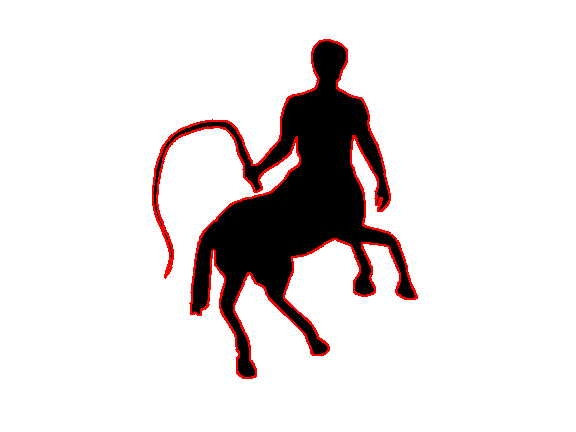## Geodesic Distance¶

We consider the geodesic distance obtained by contraining the shortest curve to be inside $\Ss$ $$d(x,x') = \umin{ \ga } \int_0^1 \abs{\ga'(t)} d t,$$ where $\ga$ should satisfy $$\ga(0)=x, \quad \ga(1)=x' \qandq \forall t \in [0,1], \: \ga(t) \in \Ss.$$

The geodesic distance map $U(x) = d(x,x_0)$ to a starting point $x_0$ can be computed in $O(q^2 \log(q))$ operations on a grid of $q \times q$ points using the Fast Marching algorithm.

Design a constraint map for the Fast-Marching, to enforce the propagation inside the shape.

In :
S1 = perform_convolution(S, ones(3)/9)<.01; S1=double(S1==0);
C = zeros(q)-Inf; C(S1==1) = +Inf;
options.constraint_map = C;


Shortcut to compute the geodesic distance to some point $x$.

In :
geod = @(x)perform_fast_marching(ones(q), x, options);


Compute the geodesic distance to a point $x$

In :
x = [263; 55];
options.nb_iter_max = Inf;
U = geod(x);


Display the distance and geodesic curves.

In :
clf;
options.display_levelsets = 1;
options.pstart = x;
options.nbr_levelsets = 60;
U(S==0) = Inf;
display_shape_function(U, options);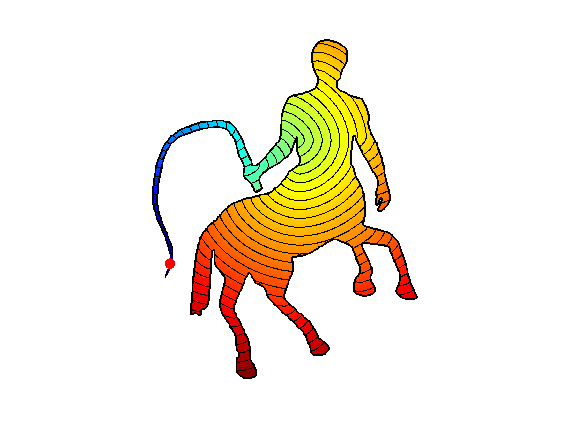Exercise 1

Compute curves joining the start point to several points along the boundary.

In :
exo1()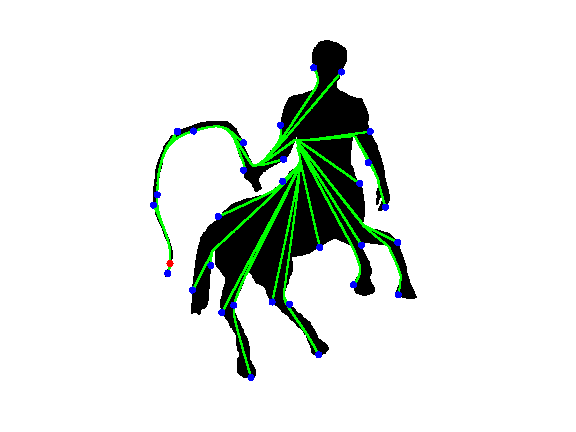In :
%% Insert your code here.


## Geodesic Distance Matrix¶

We define a set $X = (x_i)_{i=1}^n \in \RR^{2 \times N} \subset \Ss$ of sampling points.

Total number $N$ of sampling points.

In :
N = 1000;


The sampling is made of $N_0$ points on the boundary $b$, and $N-N_0$ points inside the shape $\Ss$.

Number of points on the boundary.

In :
N0 = round(.4*N);


Sampling on the boundary.

In :
I = round(linspace(1,L+1,N0+1));
X = round(b(:,I(1:end-1)));


Add $N-N_0$ points inside the shape.

In :
[y,x] = meshgrid(1:q,1:q);
I = find(S==1);
I = I(randperm(length(I))); I = I(1:N-N0);
X(:,end+1:N) = [x(I),y(I)]';


Display the sampling points.

In :
clf; hold on;
imageplot(1-S);
plot(X(2,:), X(1,:), 'r.', 'MarkerSize', 15);
axis('ij');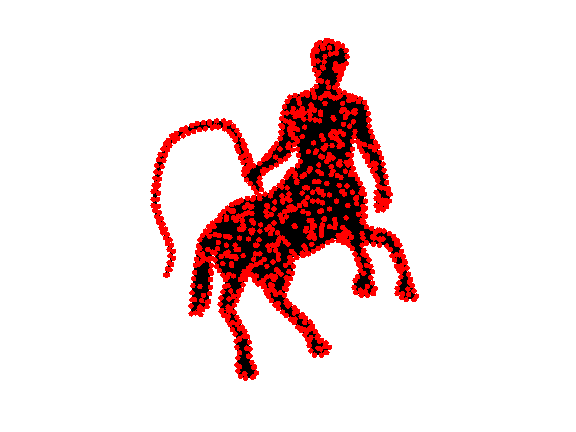The geodesic distance matrix $\de \in \RR^{N \times N}$ is defined as $$\forall i,j=1,\ldots,N, \quad \de_{i,j} = d(x_i,x_j).$$

Exercise 2

Compute the geodesic distance matrix $\de$.

In :
exo2()

In :
%% Insert your code here.


Display the geodesic matrix for $1 \leq i,j \leq N_0$ (points along the boundary $b$).

In :
clf;
imageplot(delta(1:N0,1:N0));
colormap(jet(256));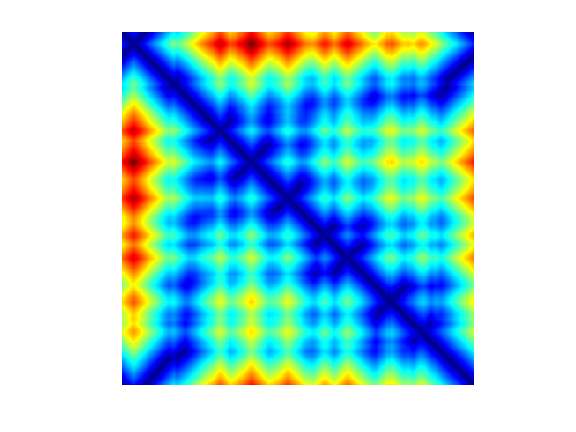## Bending Invariant with Strain Minimization¶

The goal is to compute a set of points $Y = (y_i)_{i=1}^N$ in $\RR^d$, (here we use $d=2$) stored in a matrix $Y \in \RR^{d \times N}$ such that $$\forall i, j, \quad D^2(Y)_{i,j} \approx \de_{i,j}^2 \qwhereq D^2(Y)_{i,j} = \norm{y_i-y_j}^2.$$

This can be achieved by minimzing a $L^2$ loss $$\umin{Y} \norm{ D^2(Y)-\de^2 }^2 = \sum_{i<j} \abs{ \norm{y_i-y_j}^2 - \de_{i,j}^2 }^2.$$

Strain minimization consider instead the following weighted $L^2$ loss (so-called strain) $$\umin{Y \in \RR^{d \times N} } \text{Strain}(Y) = \norm{ J ( D^2(Y)-\de^2 ) J }^2$$ where $J$ is the so-called centering matrix $$J_{i,j} = \choice{ 1-1/N \qifq i=j, \\ -1/N \qifq i \neq j. }$$

In :
J = eye(N) - ones(N)/N;


Using the properties of squared-distance matrices $D^2(Y)$, one can show that $$\norm{ J ( D^2(Y)-\de^2 ) J }^2 = \norm{ Y Y^* - K }^2 \qwhereq K = - \frac{1}{2} J \de^2 J.$$

In :
K = -1/2 * J*(delta.^2)*J;


The solution to this (non-convex) optimization problem can be computed exactly as the rows of $Y$ being the two leading eigenvectors of $K$ propery rescaled.

In :
opt.disp = 0;
[Y, v] = eigs(K, 2, 'LR', opt);
Y = Y .* repmat(sqrt(diag(v))', [N 1]);
Y = Y';


Extract the boundary part of the mapped data. Rotate the shape if necessary.

In :
theta = -.8*pi;
uv = Y(:,1:N0);
uv = [cos(theta)*uv(1,:) + sin(theta)*uv(2,:); - sin(theta)*uv(1,:) + cos(theta)*uv(2,:)];


Display the bending invariant boundary.

In :
clf;
h = plot(uv(2,:), uv(1,:));
axis('ij'); axis('equal'); axis('off');
set(h, 'LineWidth', lw);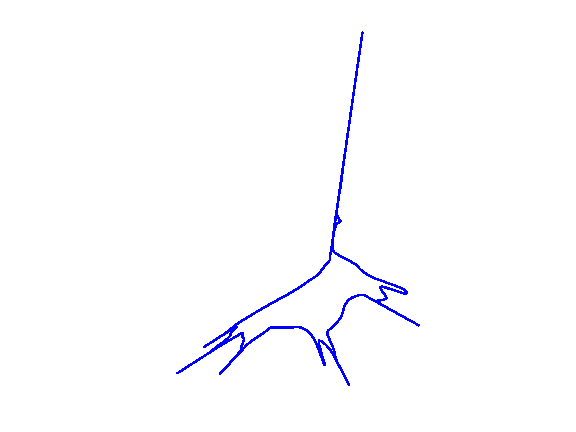## Bending Invariant with Stress Minimization¶

The stress functional does not have geometrical meaning. An alternative MDS method directly minimizes a geometric loss, the so-called Stress $$\umin{Y \in \RR^{d \times N} } \text{Stress}(Y) = \norm{ D(Y)-\de }^2 = \sum_{i<j} \abs{ \norm{y_i-y_j} - \de_{i,j} }^2.$$ It is possible to find a local minimizer of this energy by various descent algorithms, as initially proposed by Kruskal64

In :
Stress = @(d)sqrt( sum( abs(delta(:)-d(:)).^2 ) / N^2 );


Operator to compute the distance matrix $D(Y)$.

In :
D = @(Y)sqrt( repmat(sum(Y.^2),N,1) + repmat(sum(Y.^2),N,1)' - 2*Y'*Y);


The SMACOF (Scaling by majorizing a convex function) algorithm solves at each iterations a quadratic energy, that is guaranteed to diminish the value of the Strain. It was introduced by Leeuw77

It computes iterates $X^{(\ell)}$ as $$X^{(\ell+1)} = \frac{1}{N} X^{(\ell)} B(D(X^{(\ell)}))^*,$$ where $$B(D) = \choice{ -\frac{\de_{i,j}}{D_{i,j}} \qifq i \neq j, \\ -\sum_{k} B(D)_{i,k} \qifq i = j. }$$

Initialize the positions for the algorithm.

In :
Y = X/q;


Operator $B$.

In :
remove_diag = @(b)b - diag(sum(b));
B = @(D1)remove_diag( -delta./max(D1,1e-10) );


Update the positions.

In :
Y = Y * B(D(Y))' / N;


Exercise 3

Perform the SMACOF iterative algorithm. Save in a variable |s(l)| the values of Stress$( X^{(\ell)} )$.

In :
exo3()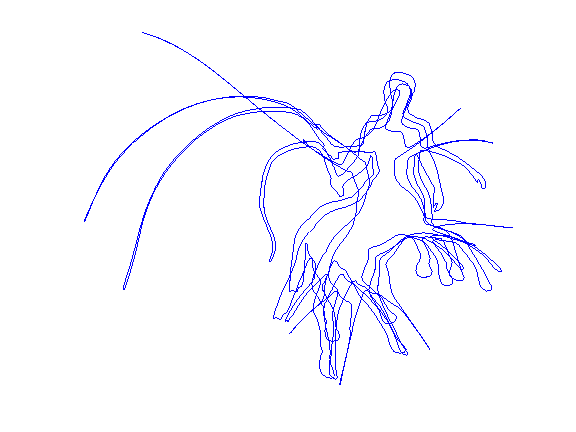In :
%% Insert your code here.


Plot stress evolution during minimization.

In :
clf;
plot(s, '.-', 'LineWidth', 2, 'MarkerSize', 20);
axis('tight');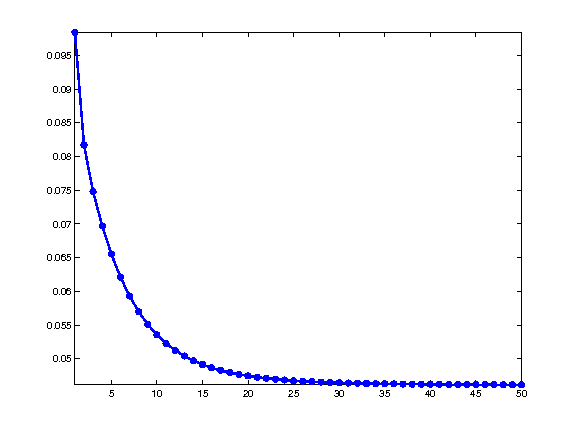Plot the optimized invariant shape.

In :
clf;
h = plot(Y(2,[1:N0 1]), Y(1,[1:N0 1]));
axis('ij'); axis('equal'); axis('off');
set(h, 'LineWidth', lw);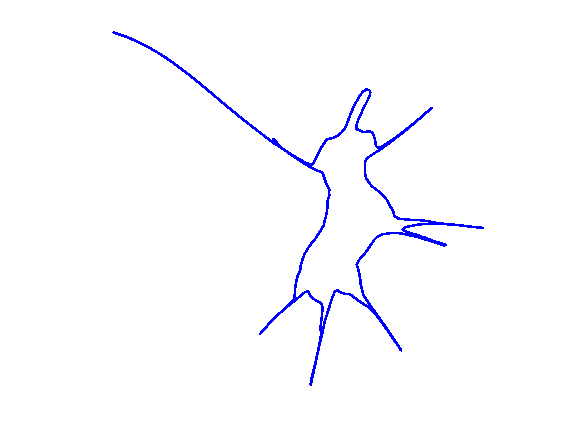## Shape Retrieval with Bending Invariant.¶

One can compute a bending invariant signature for each shape in a library of images.

Isometry-invariant retrival is then perform by matching the invariant signature.

Exercise 4

Implement a shape retrival algorithm based on these bending invariants. o correction for this exercise.

In :
exo4()

In :
%% Insert your code here.